# Marked Correlation Function¶

In the analysis of galactic conformity, we make use of the marked correlation function (MCF, see Skibba et al (2006) for more information).

The MCF has the format of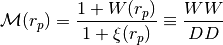where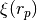is the usual two-point correlation function with pairs summed up in bins of projected separation,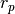, and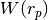is the same except that galaxy pairs are weighted by the product of their marks. The estimator used in the equation can also be written as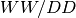, where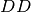is the raw number of galaxy pairs separated byand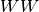is the weighted number of pairs. In the conformity analysis using MCF, we normalize by the mean of the galaxy property and then compute the MCF results.